# Infosys Reasoning Ability Previous Year Questions

03 January 2023

Infosys Reasoning Ability previous year questions

Infosys Reasoning Ability previous year questions

Q1) In the class interval 13-23, 23 is known as ___.

A. Frequency

B. Class width

C. Upper class limit

D. Lower class limit

Explanation: Class interval is 13- 23 , 13 is called as lower class limit and 23 as upper class limit, hence option C is correct answer.

Q2) For the class interval 72 - 93, the class width is ______.

A. 20

B. 21

C. 93

D. 72

Explanation: Class width = upper class - lower class = 93 - 72 = 21.

Q3) The question below consists of a question and two statements numbered I and II given, You  have to decide whether the data provided in which of the statements are sufficient to answer the question.

Z is in which direction with respect to T?

I) T is south of A & south east of B.

II) Z is east of B & A.

A. The data in statement I alone is sufficient to answer the question while the data in statement II alone is not sufficient to answer the question.

B. The data in statement II alone is sufficient to answer the question while the data in statement I alone is not sufficient to answer the question.

C. The data either in statement I alone or in statement II alone is sufficient to answer the question.

D. The data in both statements I and II together is necessary to answer the question.

Explanation: Considering data from both the statements Z is north-east direction of T.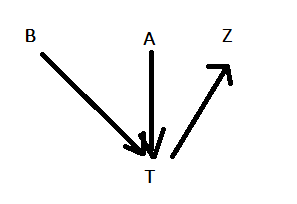Q4) Direction: The question below consists of a question and two statements numbered I and II given. You  have to decide whether the data provided in which of the statements are sufficient to answer the question.  Choose your answer from the options based on this.

What is the code for the word ‘COLD’?.

I. The code for the word ‘ROLE’ is 18,15,12,5.

II. The code for the word ‘ART’ is 1,18,20.

A. The data in statement I alone is sufficient to answer the question while the data in statement II alone is not sufficient to answer the question.

B. The data in both statements I and II together is necessary to answer the question.

C. The data in statement II alone is sufficient to answer the question while the data in statement I alone is not sufficient to answer the question.

D. The data either in statement I alone or in statement II alone is sufficient to answer the question.

Explanation: Using statement one - The code for the word ‘ROLE’ is 18,15,12,5, the code is very clear alphabet position number was taken as a code hence the code for COLD will be 3,15,12,4

Using statement two - The code for the word ‘ART’ is 1,18,20, the code is very clear alphabet position number was taken as a code hence the code for COLD will be 3,15,12,4

Hence, either of the statements alone are sufficient to identify the answer.

Q5) The range of the data 7, 10, 6, 72, 83, 60, 12, 5 is ___.

A. 78

B. 85

C. 72

D. 83

Explanation: Range = Highest observation – lowest observation = 83 – 5 = 78

Q6) In the question below, three statements are given, followed by two conclusions, I and II. You have to  consider the statement to be true even if it seems to be at variance from commonly known facts. You have to decide which of the given conclusions, if any, follows from the given statements.

Statements:

All shoes are legs. Some legs are pairs.

Some pair are hands.

Conclusions:

I. Some hands are shoes.

II. Some pairs are shoes.

A. Only conclusion II follows.

B. Only conclusion I follows.

C. Both conclusion I and II follow.

D. Neither conclusion I nor II follow.

Explanation: As per the Venn diagram, both doesn’t follow hence option D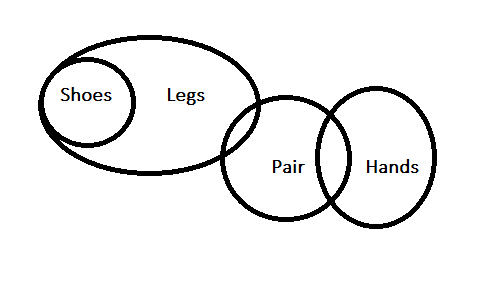Q7) Direction: The question below consists of a question and two statements numbered I and II given. You  have to decide whether the data provided in which of the statements are sufficient to answer the question. Choose your answer from the options based on this.

Among persons S, T, U, V, W and B who is the tallest?

I. U is taller than only two persons. T is taller than S but not the tallest. W is taller than only B.

II. S is shorter than only two persons. W is taller than B but shorter than U. U is shorter than S. T is shorter than V.

A. The data in statement I alone is sufficient to answer the question while the data in statement II alone is not sufficient to answer the question.

B. The data in statement II alone is sufficient to answer the question while the data in statement I alone is not sufficient to answer the question.

C. The data either in statement I alone or in statement II alone is sufficient to answer the question.

D. The data in both statements I and II together is necessary to answer the question.

Explanation: From statement I, U is taller than only two persons. T is taller than S but not the tallest. W is taller than only B.

V>T>S>U>W>B

V is the tallest

From statement II. S is shorter than only two persons. W is taller than B but shorter than U. U is shorter than S. T is shorter than V.

V>T>S>U>W>B

V is the tallest

Hence, either of the statements alone are sufficient to identify the answer.

Q8) In the question below, two statements are given, followed by two conclusions, I and II. You have to consider the statement to be true even if it seems to be at variance from commonly known facts. You have to decide which of the given conclusions, if any, follows from the given statements.

Statements:

No car is green.

Some trains are green.

Conclusion:

I. No green is car.

II. Some trains are car.

A. Both conclusion I and II follow.

B. Neither conclusion I nor II follow.

C. Only conclusion I follows.

D. Only conclusion II follows.

Explanation: As per the Venn diagram, only I follow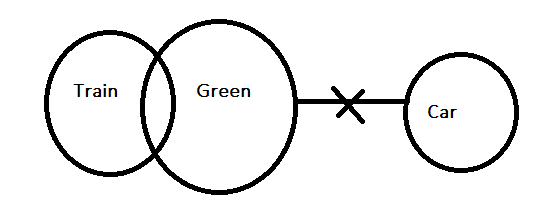Q9) Direction: The question below consists of a question and two statements numbered I and II given, You have to decide whether the data provided in which of the statements are sufficient to answer the question. Choose your answer from the options based on this.

How is C related to D?

I. A is the only son of C who is the father of E.

II. E is the sister of A and mother of D.

A. The data in statement I alone is sufficient to answer the question while the data in statement II alone is not sufficient to answer the question.

B. The data in statement II alone is sufficient to answer the question while the data in statement I alone is not sufficient to answer the question.

C. The data in both statements I and II together is necessary to answer the question.

D. The data either in statement I alone or in statement II alone is sufficient to answer the question.

Explanation: From statement I. A is the only son of C who is the father of E.

C is the father of A and E, relation between C and D can’t be found

From statement  II. E is the sister of A and mother of D.

E is the mother of D

Combining both E is the mother of D and C is father of E , hence C is grandfather of D

Hence, the data in both statements I and II together is necessary to answer the question.

Q10) For the class interval 72-93, the class width is ___.

A. More than one

B. Zero

C. One

D. Less than one

Explanation: Class width = upper class - lower class = 93 - 72 = 21, more than one

Q11) In the question below, two statements are given, followed by two conclusions, I and II. You have to  consider the statement to be true even if it seems to be at variance from commonly known facts. You have to decide which of the given conclusions, if any, follows from the given statements.

Statements:

Some A are B.

No B is C.

Conclusions:

I) Some B are C.

II) All A being C is a possibility.

A. Both conclusion I and II follow.

B. Neither conclusion I nor II follow.

C. Only conclusion I follows.

D. Only conclusion II follows.

Explanation: As per Venn diagram conclusion I is wrong, conclusion II is also not possible as little part of A should Meet with BQ12) Direction: The question below consists of a question and two statements numbered I and II given, You  have to decide whether the data provided in which of the statements are sufficient to answer the question. Choose your answer from the options based on this.

Six friends, L, M, N, O, P and Q are sitting in a row facing north. What is the position of Q with respect to the position of P?

I. L is sitting fourth to the right of M whose only neighbour is Q.

II. O is sitting second to the right of P.

A. The data in statement I alone is sufficient to answer the question while the data in statement II alone is not  sufficient to answer the question.

B. The data in statement II alone is sufficient to answer the question while the data in statement I alone is not sufficient to answer the question.

C. The data either in statement I alone or in statement II alone is sufficient to answer the question.

D. The data in both statements I and II together is necessary to answer the question.

Explanation:

1) L is sitting fourth to the right of M whose only neighbour is Q.

As Q is the only neighbour of M, implies, M is sitting on the leftmost end.

M,Q,_,_,L,_

2) O is sitting to the right of P.

Implies, O is sitting on the rightmost end as P is sitting on the fifth seat from left.

Considering both the order will be

M,Q,N,P,L,O

The data in both statements I and II together is necessary to answer the question

Q13) Frequency of any observation can’t be ___.

A. 3

B. 2.5

C. 22

D. 5

Explanation: Frequency can’t be in decimals, hence option B

Q14) Please select the right set of logically related combination :

A. Some painters are farmers, and some are not.

B. Raman is a farmer.

C. All painters are choreographers.

D. Raman its a painter.

E. Some farmers are choreographers.

B. CDE

C. ACE

D.  DAB

Explanation: Consider 1st and 2nd Term as the statement and 3rd should be the conclusion

Going through option verification only option D satisfy, A and C are the statements and E is the conclusion.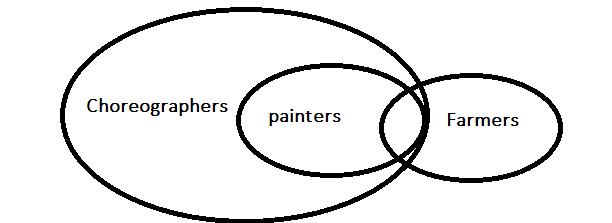Q15) In the question below, two statements are given, followed by two conclusions, I and II. You have to  consider the statement to be true even if it seems to be at variance from commonly known facts. You have to decide which of the given conclusions, if any, follows from the given statements.

Statements:

Some Apples are Mangoes.

All Mangoes are Bananas.

Conclusion:

I. All Apples are Bananas.

II. Some Apples are not Bananas.

A.  Only conclusion II follows.

B.  Only conclusion I follows.

C.  Both conclusion I and II follow.

D. Either I or II follows.

Explanation: As per the Venn diagram some Apples are not bananas, when some are not bananas, all case is not possible, there is a possibility that all apples can be inside mango and all apples can be banana when all is possible some not will not happen , hence either I or II follows.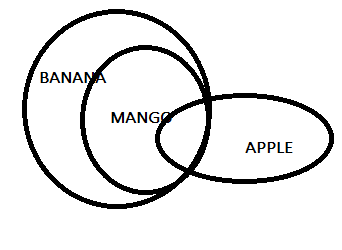Q16) What is the area of triangle ABC?

Statements:

A. AB = 5 cm, BC = 6 cm, AC = 11 cm

B. Angle C < 900

A. If statement A alone is sufficient to answer the question.

B. If statement B alone is sufficient to answer the question.

C. If both statements together are sufficient to answer the question but neither statement alone is sufficient.

D. If neither A nor B is sufficient to answer the question.

E. If either A alone or B alone is sufficient to answer the question.

Explanation: From statement A, all sides are given the area of the triangle can be found by using the sides

Area of triangle = √[s(s – a)(s – b)(s – c)],formulae can be used

By using statement B, only with the help of one angle area can’t be found out .

Hence option A is correct answer.

Q17) Directions: The question contains six statements followed by four sets of combinations of three. Choose the set in which the statement are logically related.

A. Some people are corrupt.

B. Power corrupt with time.

C. He is powerful.

D. Corrupt people have power.

E. He is corrupt.

F. Some people have power

A. CBE

B. ECB

Explanation: Consider 1st and 2nd Term as the statement and 3rd should be the conclusion

Going through option verification only option D satisfy, A and D are the statements and F is the conclusion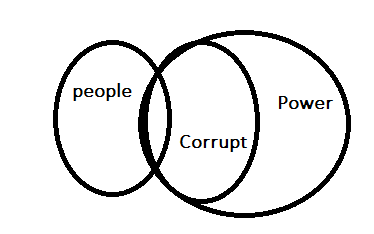Q18) Please select the right set of logically related combination :

A. Some fishes are not carnivorous.

B. All fishes are swimmers.

C. All fishes are carnivorous.

D. Some swimmers are not carnivorous.

E. Some swimmers are carnivorous.

A) DCB

B) EDA

C) BEC

D) None of the above

Explanation: Consider 1st and 2nd Term as the statement and 3rd should be the conclusion

Going through option verification none of the options follows.

Q19) Directions: The question below has a few statements, followed by four conclusions every given statements as true , even if it does not conform to the well known facts.

Statements:

1.Some birds are mangoes.

2.No duck is a parrot.

3.All mangoes are ducks.

Conclusion:

I.Some birds are duck

II.Some ducks are mangoes.

III.No parrot is duck.

IV.Some birds are not parrots.

A. Only I, II and III follow

B. Only II, III and IV follow

C. Only I, III and IV follow

D. All follow

E. Only I, II, IV follow

Explanation: As per Venn diagram all conclusions follow.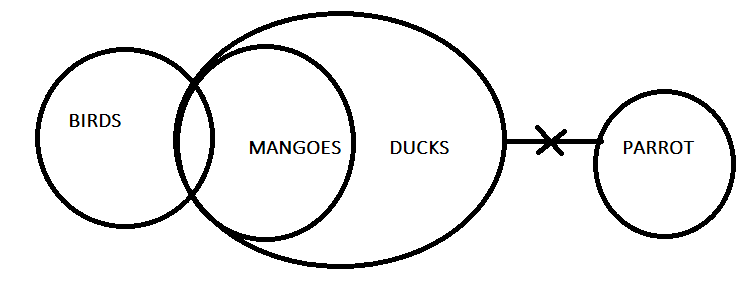Q20) Directions: The problem has a question followed by two statements which are marked I and II. Use the data given in A and B together or separately and mark.

What is the value of p – 2q?

I. p – 3q = 4

II. p> 0 , q > 0

A. Only I alone sufficient

B. Only II alone sufficient

C. Both the statements are sufficient

D. Cannot be determined

Explanation: From statement I. p – 3q = 4

Possible values of P and Q are

P       Q

10     2

13      3

16      4

And soon on, finding exact value of P and Q is not possible.

From II. p> 0, q > 0, all +ve numbers are greater than 0 and can take the value of P and Q.

Consider both statements also multiple answers are possible, hence can’t determine.

##